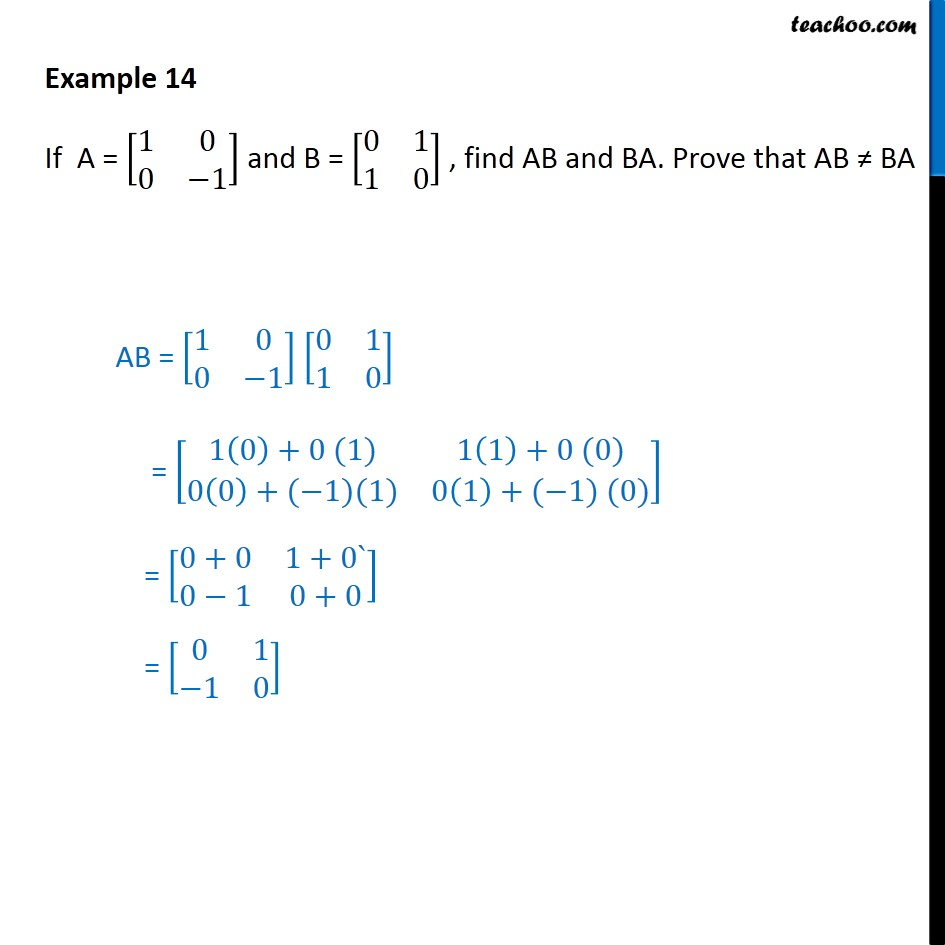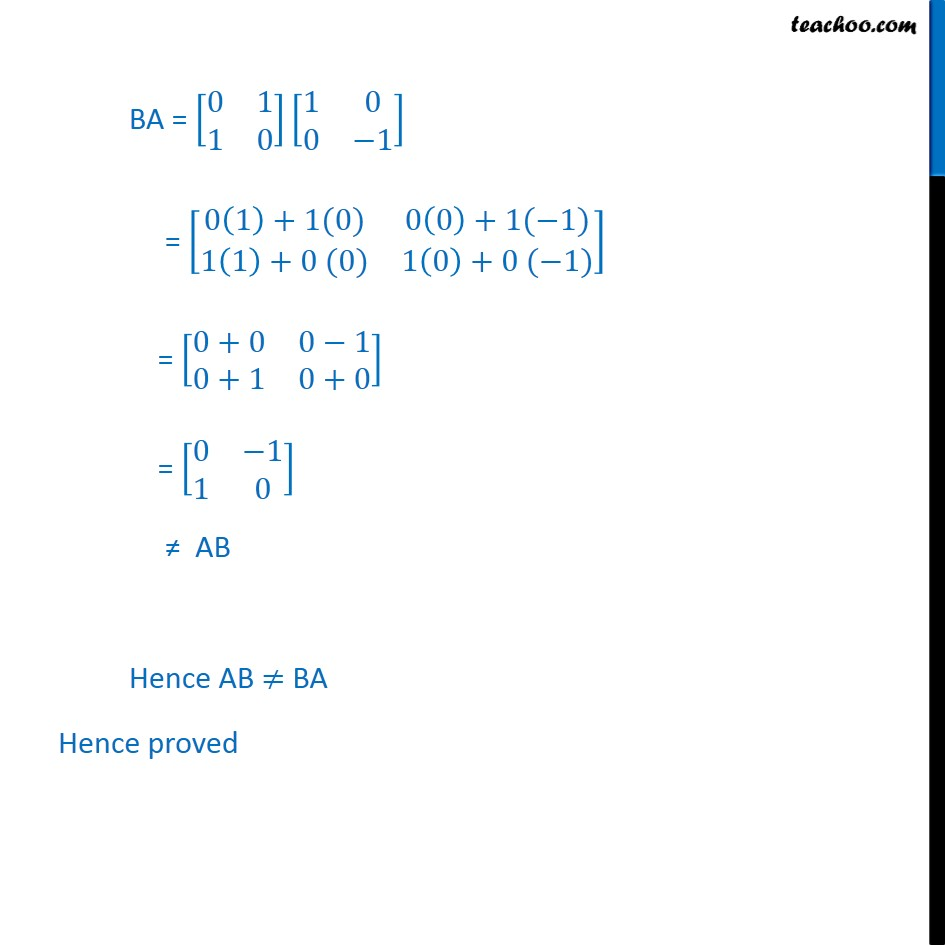1. Chapter 3 Class 12 Matrices
2. Concept wise
3. Multiplication of matrices

Transcript

Example 14 If A = [■8(1&0@0&−1)] and B = [■8(0&1@1&0)] , find AB and BA. Prove that AB ≠ BA AB = [■8(1&0@0&−1)] [■8(0&1@1&0)] = [■8(1(0)+0 (1)&1(1)+0 (0)@0(0)+(−1)(1)&0(1)+(−1) (0))] = [■8(0+0&1+0`@0−1&0+0)] = [■8(0&1@−1&0)] BA = [■8(0&1@1&0)][■8(1&0@0&−1)] = [■8(0(1)+1(0)&0(0)+1(−1)@1(1)+0 (0)&1(0)+0 (−1))] = [■8(0+0&0−1@0+1&0+0)] = [■8(0&−1@1&0)] ≠ AB Hence AB ≠ BA Hence proved

Multiplication of matrices